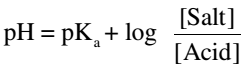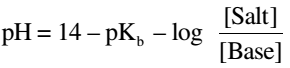# Change In pH By Common Ion Effect Using Universal Indicator Solution or pH Paper

Weak acids and bases do not dissociate completely. An equilibrium exists between the dissociated and un-dissociated molecules.

HA ⇌ H+ + A-

This equilibrium is characterized by a constant, Ka. Addition of a salt containing a common ion, say NaA to its solution causes the equilibrium to shift to the left (Le Chatelier’s principle).

As a consequence, the concentration of hydrogen ions gets reduced and the pH gets increased.

Such mixtures of weak acid or weak bases with their respective salts are called as Buffer Solution. pH of an acidic buffer can be calculated by using the following equation:Ka is the acid dissociation constant and pKa is the negative logarithm of Ka.

pH for a basic buffer (a weak base and its salt) can be calculated by using the following equation:Kb is the base dissociation constant and pKb is the negative logarithm of Kb.

pKa for acetic acid at 25°C = 4.76

pKb for ammonium hydroxide at 25°C = 4.75

Experiment: To study the pH change by common ion effect in case of weak acids and weak bases using universal indicator solution or pH paper.

### How To Perform Experiment

Take 5 labelled test tubes and place them in a test tube stand. Take 5 mL of given acetic acid solution in each of the labelled test tubes. Add 0.5 g, 1.0 g, 1.5 g and 2.0 g of sodium acetate to test tube No. 2, 3, 4 and 5 respectively and dissolve to get clear solutions.

Add 4-5 drops of the universal indicator solution to each of the test tubes. In case of pH paper, add 1 -2 drops of the solutions to pH papers separately with the help of separate dropper. Alternatively, you may dip the pH paper strips into each of the test solution.

Compare the colours in test solution or on pH paper developed with the colour chart and record the corresponding pH values. Calculate the pH of each buffer solution you have prepared using the appropriate equation.

You can try the same procedure to study the pH in case of basic buffer using ammonium hydroxide as a base and ammonium chloride as its salt.